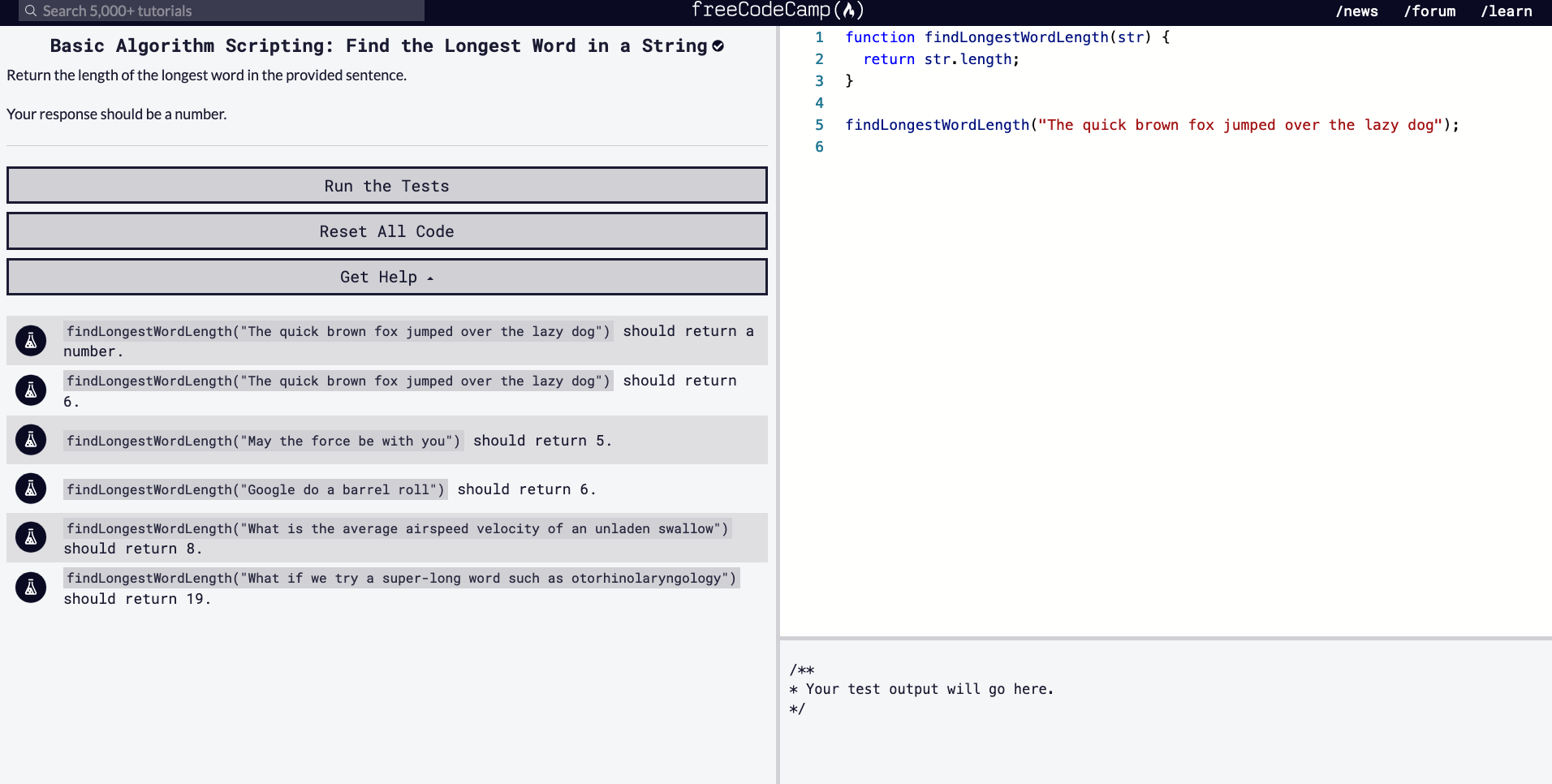# FCC Algorithm Challenges / Find the Longest Word in a String

### 5/1/2020``````function findLongestWordLength(str) {
return str.length;
}

findLongestWordLength("The quick brown fox jumped over the lazy dog");

/// TESTS
findLongestWordLength("The quick brown fox jumped over the lazy dog") should return a number.
findLongestWordLength("The quick brown fox jumped over the lazy dog") should return 6.
findLongestWordLength("May the force be with you") should return 5.
findLongestWordLength("Google do a barrel roll") should return 6.
findLongestWordLength("What is the average airspeed velocity of an unladen swallow") should return 8.
findLongestWordLength("What if we try a super-long word such as otorhinolaryngology") should return 19.
``````

Above is the starter code provided for the challenge, "Find the Longest Word in a String."

Our goal is to write a function that to take the input of a string and return its the longest word's length contained in that string (so we want to return an integer). Let's think this through. Here is how I would aim to solve this problem.

## Method

• Read the instructions first. Make sure you understand what it being asked of you.
• Read the starter code. Go line by line, just making sure you know what is going on initially.
• Have a look at the tests. If the problem isn't clear to you, looking at the tests might give you an inclination of what kind of output you should aim for (i.e. instead of returning an array, maybe the problem is only asking for an index within the array).
2. Think & Write

Now that you've read through the instructions, starter code, and tests, it's time to analyze what to do and in what order. It may be handy to write out pseudocode.

3. Code

Once you've thought about what you'd like to do, and in what order, start to convert your pseudocode into JavaScript code.

There's been too many times where I've tried to jump write into writing the code without thinking it through (in projects and coding challenges). That will leave you testing it way too many times, creating unnecessary variables, and running into more problems then you need to handle. If I try to follow the above method, it leaves me with a more clear mind of what I'm doing and hopefully writing some DRY code. Let's try to solve this problem now.

## Thoughts

• The inputs from the tests are sentences, a string of words
• The string data type has a property, `.length` which will return the number of characters within that string (i.e. `'hello'.length // returns 5`)
• The `.length` property is helpful but the inputs are sentences, so we have to go through each word and check the length
• Like an earlier problem, it might be a good idea to split the string into an array, each word stored in its own index, using `str.split(' ')`
• It will also be a good idea to declare a new variable, which we will use to compare the string lengths against, `let longest = 0;`
• Our `str` input is now an array, we can loop through each index, and check the length of each word
• We will set the initial word's length to the `longest`, then we can use an if statement, if the next word's length is longer, it can be set to the `longest` variable
• Make sure to return something, we want to return `longest`, which should hopefully give us an integer of the longest word's length

## Solution

Some Pseudocode

``````function findLongestWordLength(str) {
set variable to hold longest length
split str input into an array
loop through the new str array
check if  value in str array is greater than longest
set str array value to longest
(will repeat until we go through every value in array)
return longest;
}
``````
**[SPOILER: SOLUTION TO CODE BELOW]** ```javascript function findLongestWordLength(str) { let longest = 0; let strArray = str.split(' '); for (let word of strArray) { if (word.length > longest) { longest = word.length; } } return longest; } ```

We can also use a for loop instead of for .. of loop.

``````function findLongestWordLength(str) {
let longest = 0;
let strArray = str.split(' ');
for (let i = 0; i < strArray.length; i++) {
if (strArray[i].length >= longest) {
longest = strArray[i].length;
}
}
return longest;
}
``````